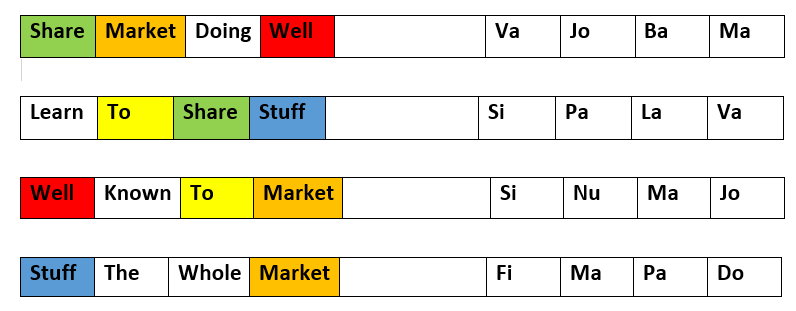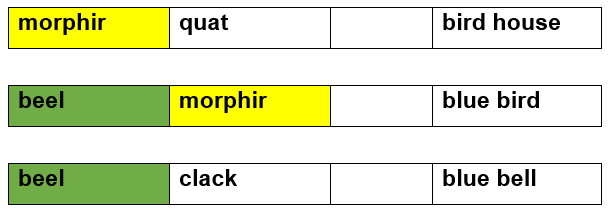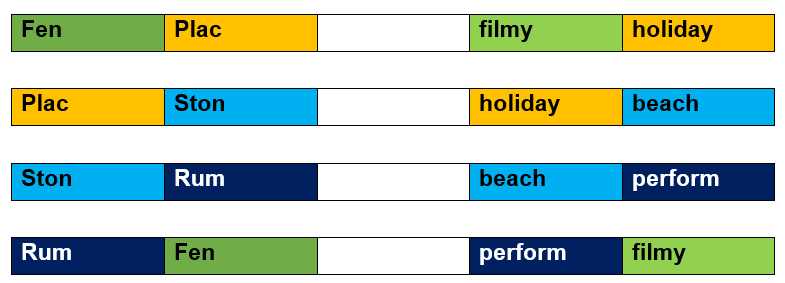# Artificial Language

## Introduction

Artificial Language is a human-made pattern that aims to imitate humans' logical reasoning process in machines. Artificial language is similar to human-readable language.

This language is created from the existing letters from different languages such that those can be related to each other through some pattern or relation.

This topic contains questions commonly asked in various tests and has a significant role in achieving high marks.

## Characteristics of Artificial Language

1. Artificial language is a constructed or invented language.
2. It does not have any grammar, phonics or anything similar to natural languages.
3. The problem structure of the artificial language topic is given below.

## Problem Structure

Some English words are given with their translation in a hypothetical language. The question requires us to predict how a given word will look like in that language. The skill needed here is pattern recognition.

## Tips For Solving the Problem of Artificial Language

Steps for solving the problem of artificial language are as follows:

1. We can't learn all of the artificial languages by their proper meanings, therefore don't attempt.

1. Understanding the examples is sufficient for answering this type of question.

1. At times, the question will be challenging to answer. There could be a grammatical error in the question. So, write artificial language independently and then solve the problem for each word in the provided example.

## Problems related to Artificial Language

### Type 1 Problems

Problem 1:

The problem states that: ‘Share market doing well’ is written as ‘Va Jo Ba Ma’‘learn to share stuff’ is written as ‘Si Pa La Va’‘well known to market’ is written as ‘Si Nu Ma Jo’ and ‘stuff the whole market’ is written as ‘Fi Ma Pa Do’. What does the code ‘Ba’ stand for?

Solution:

The above question can be diagrammatically represented as follows:• In the first two rows, Share is common; The common translation in those rows is Va.

Which means Share is translated as Va.

• In the second and third-row To is common; The common translation in those rows is Si.

Which means To is translated as Si.

• In the first, third and last row, Market is common; The common translation in those rows is Ma.

Which means Market is translated as Ma.

• In the first and third row, Well is common; The common translation in those rows is Jo.

Which means Well is translated as Jo.

Now, from the first row, we can conclude that Doing is translated as Ba.

Problem 2: In a particular language, morpir quat means birdhouse, beel morpir means bluebird and beel clak means bluebell. Which word could mean "houseguest"?

Solution:

The above question can be diagrammatically represented as follows:From the 1st and 2nd rows, we can conclude that morphir is translated as a bird.

From the 2nd and 3rd rows, we can conclude that beel is translated as blue.

From the 1st row, we can figure out that quat is translated as a house.

So, the houseguest must start from quat, and the correct option is 3.

Problem 3: In a particular language, FenPlac means filmy holiday, PlacSton means holiday beach, StonRum means beach perform, and RumFen means perform filmy. Which word means “holiday”?

Solution:

The above question can be diagrammatically represented as follows:From the first row in the above diagram, we can see that Plac is translated as holiday.

### Type 2 Problems

Problem 1: If LETTER is written as ERLETT in another language. Then in the same language, what will be written for BUTTER?

Solution: In this particular language, BUTTER will be written as ERBUTT. Because LETTER is written as ERLETT, only the last two letters are added first.

Now let’s move on to the frequently asked questions.

Question 1: What is Artificial Language?

Answer: Artificial language is created from the existing letters in the language such that two languages can be related to each other through some pattern or relation.

Question 2: How to solve the problem of Artificial language?

Answer: The steps in the solution of the artificial language problem are:

• Break the word into smaller words into an artificial language
• Remove incorrect translation or incorrect order in the appearance of the word.

Question 3: Is the Venn Diagram helpful in solving the problem of Artificial language?

Answer: Venn diagram simplifies the problem, so it is used in the problem solving of Artificial language.

## Key takeaways

In this blog, we’ve covered the problem of Artificial language with the help of the Venn diagram.

You can use CodeStudio to practise questions on artificial language and use the Coding Ninja Self-paced Aptitude Preparation course to grasp numerous Aptitude concepts.